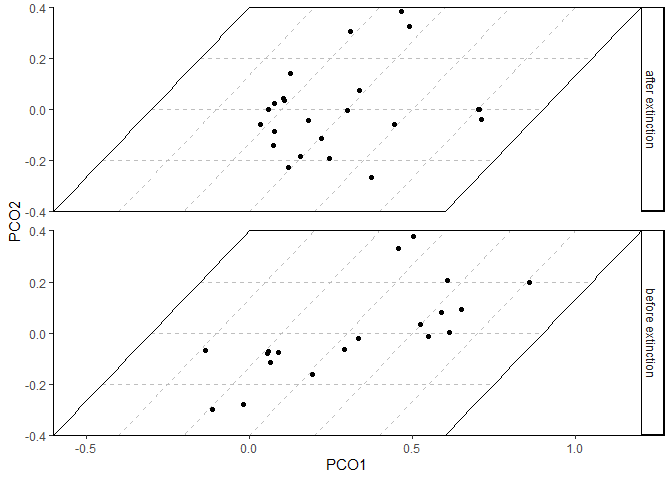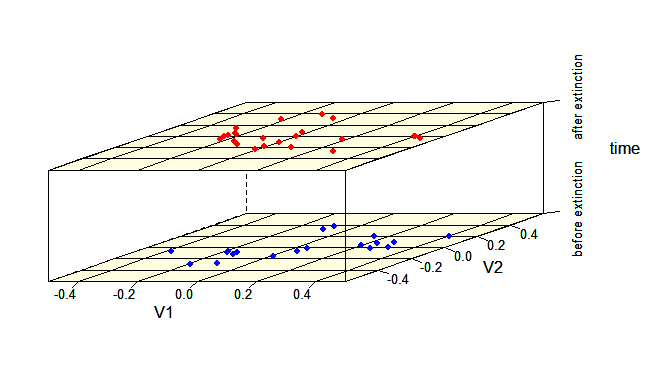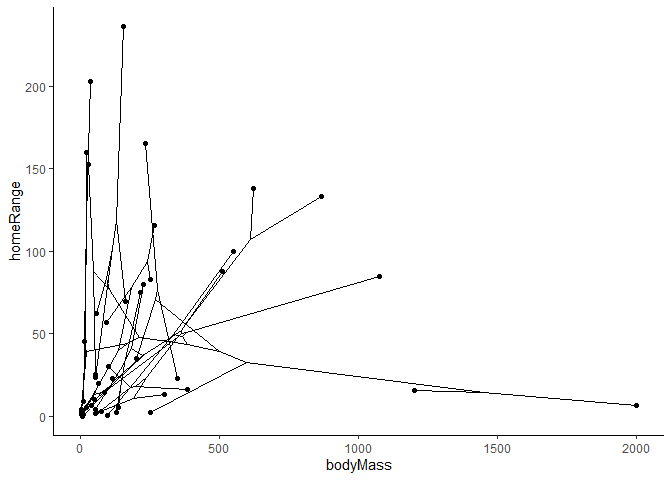# Plotting trait data

Many packages exist to visualize trait data for biological species. deeptime similarly has a few novel ways to help you plot your data in useful ways. We’ll first load some packages and example data so we can demonstrate some of this functionality.

# Load deeptime
library(deeptime)
library(ggplot2)
library(dplyr)
library(magrittr) # for piping
# Load dispRity for example data
library(dispRity)
data(demo_data)
# Load paleotree for example data
library(phytools)
data(mammal.tree)
data(mammal.data)

## Plot disparity through time

A common way to visualize trait data, especially for fossil species, is to show the two-dimensional trait distribution for several time intervals. This allows the viewer to easily compare the trait distribution through time. However, producing such a plot has historically been very time intensive, often involving the use of custom code and image editing software (e.g., Inkscape). While a single function to accomplish such a visualization still does not exist for {ggplot2} (yet…), the coord_trans_xy() function can be used to generate a similar plot with sheared trait space across several time intervals.

# make transformer
library(ggforce)
trans <- linear_trans(shear(.75, 0))

# prepare data to be plotted
crinoids <- as.data.frame(demo_data$wright$matrix[][, 1:2])
crinoids$time <- "before extinction" crinoids$time[demo_data$wright$subsets$after$elements] <- "after extinction"

# a box to outline the trait space
square <- data.frame(V1 = c(-.6, -.6, .6, .6), V2 = c(-.4, .4, .4, -.4))

ggplot() +
geom_segment(
data = data.frame(
x = -.6, y = seq(-.4, .4, .2),
xend = .6, yend = seq(-0.4, .4, .2)
),
aes(x = x, y = y, xend = xend, yend = yend),
linetype = "dashed", color = "grey"
) +
geom_segment(
data = data.frame(
x = seq(-.6, .6, .2), y = -.4,
xend = seq(-.6, .6, .2), yend = .4
),
aes(x = x, y = y, xend = xend, yend = yend),
linetype = "dashed", color = "grey"
) +
geom_polygon(data = square, aes(x = V1, y = V2), fill = NA, color = "black") +
geom_point(data = crinoids, aes(x = V1, y = V2), color = "black") +
coord_trans_xy(trans = trans, expand = FALSE) +
labs(x = "PCO1", y = "PCO2") +
theme_classic() +
facet_wrap(~time, ncol = 1, strip.position = "right") +
theme(panel.spacing = unit(1, "lines"), panel.background = element_blank())### Disparity in base R

The disparity_through_time() function accomplishes nearly all of the work for you if you are comfortable plotting within the {lattice} framework (base R). Note that it may take some tweaking (especially the aspect argument) to get the results to look the way you want.

crinoids$time <- factor(crinoids$time)
disparity_through_time(time ~ V2 * V1,
data = crinoids, groups = time, aspect = c(1.5, .6),
xlim = c(-.6, .6), ylim = c(-.5, .5),
col.regions = "lightyellow", col.point = c("red", "blue"),
par.settings = list(
axis.line = list(col = "transparent"),
layout.heights =
list(
),
layout.widths =
list(
)
)
)## Phylomorphospaces

Often, trait data will be accompanied with a phylogeny. You may want to visualize both your phylogeny, the traits of your species, and the evolution of the trait along your phylogeny. To accomplish this, you can create a two-dimensional phylomorphospace. The {phytools} package has the phytools::phylomorphospace() function for accomplishing this in base R. The geom_phylomorpho() function in deeptime will help you accomplish this with ggplot(). Note that labels can be added using geom_label() or ggrepel::geom_label_repel(), but they are not demonstrated here because they would obscure the phylogenetic relationships.

mammal.data\$label <- rownames(mammal.data)

ggplot(mammal.data, aes(x = bodyMass, y = homeRange, label = label)) +
geom_phylomorpho(mammal.tree) +
theme_classic()